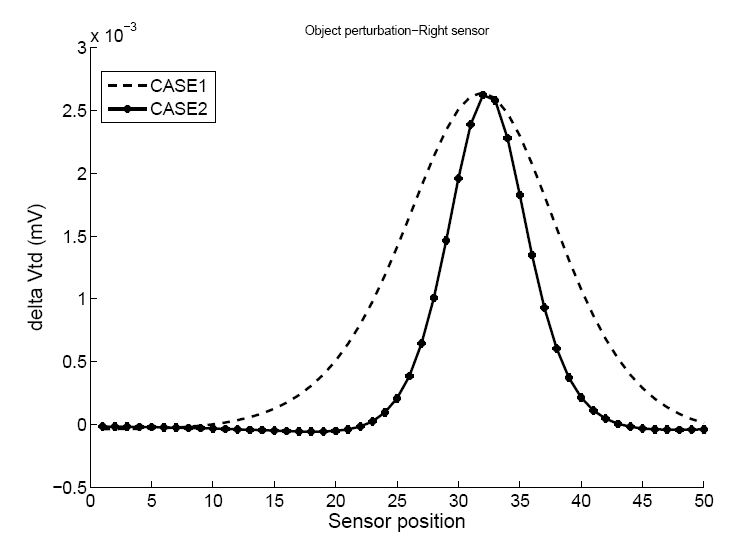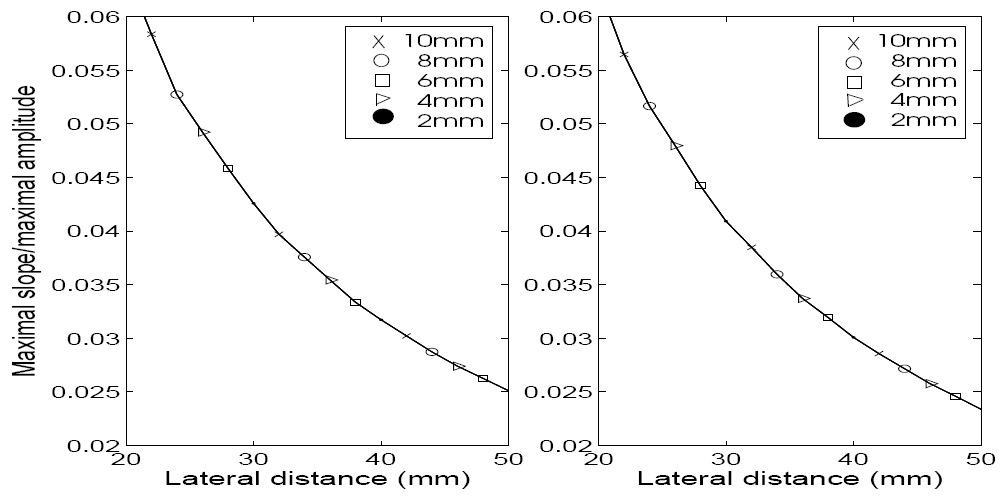﻿ Biological Cybernetics Lab

- ELECTRIC FISH

Electric fish

The figure show us the Apteronotus albifron among of weakly electric fishes.
Weakly electric fish generate electric field to navigate, detect object, and communication. There are many studies to know how electric fish identify the object with their electroreceptor. To understand the mechanism of electric fish¡¯s active electrolocation, we will use temporal electric signal with tail bending and spatial information with a lot of sensors on surface of them. This approach is expected to suggest more effective and actual neuronal structure for electrolocation.
Weakly electric fish use 'active electrolocation' that is active sensing to identify the target object by detecting distortion of self-generated electric field. The following figure is the simulation result that changes of transdermal voltage of the fish occurred by object. This result give us chance to understand how they identify the object.Figure 1. Object perturbation; (a), (c), and (e) shows how the object parameter changes, where the solid line is for the electric organ, and a circle for the target object. (a) and (b) is the result with varying rostrocaudal position, (c) and (d) with varying distances from the body axis, and (e) and (f) with varying sizes of objects

From this result suggest how weakly electric fish can identify the target object. The rostrocaudal position of the target object determines the location of maximal amplitude of a transdermal potential. The peak amplitude affected by the size and lateral distance of the target object. Next figure shows two cases that different size and lateral distance make peak amplitudes same.Figure 2. Spatial pattern of electric potential depending on object size (two differentobjects have the same peak amplitude and position; compare an object (CASE 1) with radius 1cm and distance 3.9cm, and an object (CASE 2) with radius 0.4cm and distance 2cm

However we can notice that slopes of two curves are different. Already the relative slope is known as a distance measure without consideration of size and conductivity of the target object.Figure 3. Ratio of the maximal slope/maximal amplitude (left and right curves representno tail-bending and tail-bending angle 45 degree, respectively)

Relative slope is the ratio of the maximal slope and maximal amplitude and Figure 3 shows that the relative slope is not affected by size and conductivity of the target object.
This relative slope is a spatial structure acquired from the group of a lot of electroreceptors. Together with temporal structure can be a cue to measure distance. Following figures are temporal structures with tail bending which observed at three different point.Figure 4. Temporal patterns with varying conductivities (from the electrosensor values at a distance of 4cm, 12cm, 20cm from the mouth, respectively); sphere objects with a radius of 2 cm were tested at the fixed distance of 5 cm (’'o’': positive conductivity and ’'x’': negative conductivity)Figure 5. Temporal patterns with varying sizes (from the electrosensor values at a distance of 4cm, 12cm, 20cm from the mouth, respectively); sphere objects with the conductivity 0.5 were tested at the fixed distance of 5 cm (sphere objects with varying radius sizes from 0.5cm to 2.5cm)Figure 6. Temporal patterns with varying rostrocaudal positions of an object (from the electrosensor values at a distance of 4cm, 12cm, 20cm from the mouth, respectively); the sphere object was tested at varying rostrocaudal distances of 2.4cm (solid), 3.6cm (dashed), 4.8cm (dotted), 6.0cm (dotdashed) from the mouth and at the fixed lateral distance of 5cm

Figure 4,5,6 suggest that temporal structure with tail bending can be a distance measure. In Figure 4 and Figure 5, size and conductivity is not a factor to distort the normalized tail bending pattern.